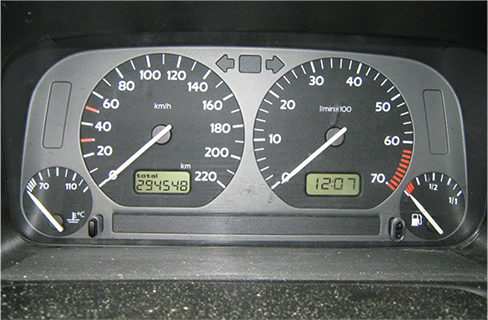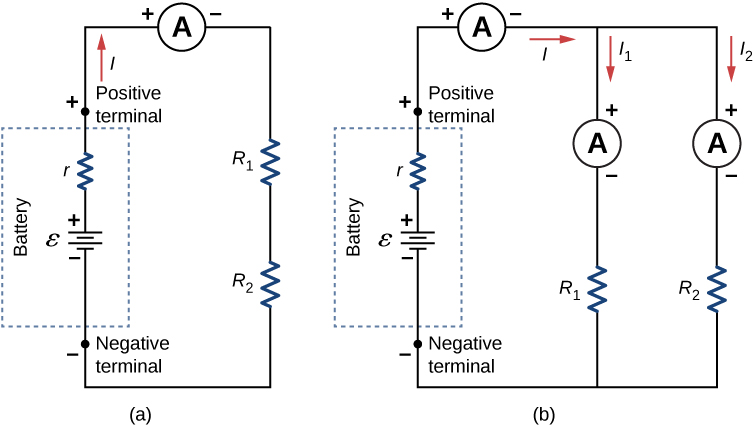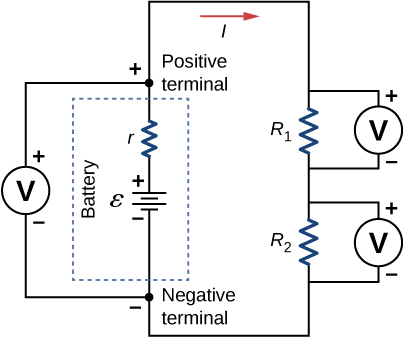# 10.4 Electrical measuring instruments

 Page 1 / 4
By the end of the section, you will be able to:
• Describe how to connect a voltmeter in a circuit to measure voltage
• Describe how to connect an ammeter in a circuit to measure current
• Describe the use of an ohmmeter

Ohm’s law and Kirchhoff’s method are useful to analyze and design electrical circuits, providing you with the voltages across, the current through, and the resistance of the components that compose the circuit. To measure these parameters require instruments, and these instruments are described in this section.

## Dc voltmeters and ammeters

Whereas voltmeter     s measure voltage, ammeter     s measure current. Some of the meters in automobile dashboards, digital cameras, cell phones, and tuner-amplifiers are actually voltmeters or ammeters ( [link] ). The internal construction of the simplest of these meters and how they are connected to the system they monitor give further insight into applications of series and parallel connections.The fuel and temperature gauges (far right and far left, respectively) in this 1996 Volkswagen are voltmeters that register the voltage output of “sender” units. These units are proportional to the amount of gasoline in the tank and to the engine temperature. (credit: Christian Giersing)

## Measuring current with an ammeter

To measure the current through a device or component, the ammeter is placed in series with the device or component. A series connection is used because objects in series have the same current passing through them. (See [link] , where the ammeter is represented by the symbol A.)(a) When an ammeter is used to measure the current through two resistors connected in series to a battery, a single ammeter is placed in series with the two resistors because the current is the same through the two resistors in series. (b) When two resistors are connected in parallel with a battery, three meters, or three separate ammeter readings, are necessary to measure the current from the battery and through each resistor. The ammeter is connected in series with the component in question.

Ammeters need to have a very low resistance, a fraction of a milliohm. If the resistance is not negligible, placing the ammeter in the circuit would change the equivalent resistance of the circuit and modify the current that is being measured. Since the current in the circuit travels through the meter, ammeters normally contain a fuse to protect the meter from damage from currents which are too high.

## Measuring voltage with a voltmeter

A voltmeter is connected in parallel with whatever device it is measuring. A parallel connection is used because objects in parallel experience the same potential difference. (See [link] , where the voltmeter is represented by the symbol V.)To measure potential differences in this series circuit, the voltmeter (V) is placed in parallel with the voltage source or either of the resistors. Note that terminal voltage is measured between the positive terminal and the negative terminal of the battery or voltage source. It is not possible to connect a voltmeter directly across the emf without including the internal resistance r of the battery.

Maxwell's stress tensor is
Yes
doris
neither vector nor scalar
Anil
if 6.0×10^13 electrons are placed on a metal sphere of charge 9.0micro Coulombs, what is the net charge on the sphere
18.51micro Coulombs
ASHOK
Is it possible to find the magnetic field of a circular loop at the centre by using ampere's law?
Is it possible to find the magnetic field of a circular loop at it's centre?
yes
Brother
The density of a gas of relative molecular mass 28 at a certain temperature is 0.90 K kgmcube.The root mean square speed of the gas molecules at that temperature is 602ms.Assuming that the rate of diffusion of a gas in inversely proportional to the square root of its density,calculate the density of
A hot liquid at 80degree Celsius is added to 600g of the same liquid originally at 10 degree Celsius. when the mixture reaches 30 degree Celsius, what will be the total mass of the liquid?
Under which topic
doris
what is electrostatics
Study of charges which are at rest
himanshu
Explain Kinematics
Two equal positive charges are repelling each other. The force on the charge on the left is 3.0 Newtons. Using your notes on Coulomb's law, and the forces acting on each of the charges, what is the force on the charge on the right?
Using the same two positive charges, the left positive charge is increased so that its charge is 4 times LARGER than the charge on the right. Using your notes on Coulomb's law and changes to the charge, once the charge is increased, what is the new force of repulsion between the two positive charges?
Nya
A mass 'm' is attached to a spring oscillates every 5 second. If the mass is increased by a 5 kg, the period increases by 3 second. Find its initial mass 'm'
a hot water tank containing 50,000g of water is heated by an electric immersion heater rated at 3kilowatt,240volt, calculate the current
what is charge
product of current and time
Jaffar
Why always amber gain electrons and fur loose electrons? Why the opposite doesn't happen?
A closely wound search coil has an area of 4cm^2,1000 turns and a resistance of 40ohm. It is connected to a ballistic galvanometer whose resistance is 24 ohm. When coil is rotated from a position parallel to uniform magnetic field to one perpendicular to field,the galvanometer indicates a charge
Using Kirchhoff's rules, when choosing your loops, can you choose a loop that doesn't have a voltage?
how was the check your understand 12.7 solved?By Yasser IbrahimBy OpenStaxBy Abishek DevarajBy Janet ForresterBy Janet ForresterByBy OpenStaxBy Yacoub JayoghliBy Jesenia WoffordBy OpenStax